# Secondary Dominants

In common practice period music (about 1650-1900) there a strong relationship between dominant and tonic. Normally, there’s only one dominant in each key. However, through some simple chromatic additions we can introduce chords into a key that function as dominants to chords other than tonic.

That is, in a V7 (dominant) chord we have two tendency tones the 7th and the 3rd. The 7th moves down and the 3rd moves up. So in C major (3rd in red, 7th in green):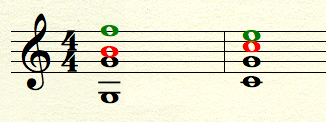Let’s examine the dominant chord. It’s a major triad (G B D) accompanied by a minor 7th (F). By copying that sort of formula, we can apply a dominant to just about any chord. But the most common way to use these so called secondary dominants is by applying them to the V chord. The result is we get a chord labled V/V–V of five. Or the dominant of the V.

Construction

In our normal dominant, the root is a fourth below or fifth above the root tonic. This is our first step. Let’s set up or temporary “tonic”: G. A fourth below or fifth above G is D. That’s our V/V chord’s root. Here’s our progression so far: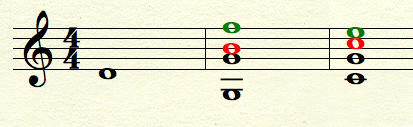The next step is to construct a dominant 7th chord built on that root. So for the D, that means we use the pitches D F# A C (a major triad: D F# A, and a minor seventh: C).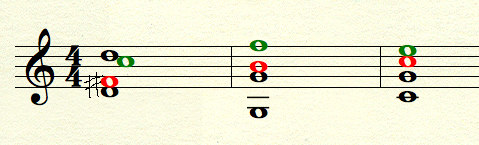So the third of V/V (D7) moves up to the root of V (G7), and the 7th of V/V moves down to the third of V. The dominant resolves as it did above.

The Process: Cliff Notes

1. Determine which chord you’d like to apply a secondary dominant to
2. Find the root of the secondary dominant a fourth below/fifth above the root of the chord to which the dominant will be applied
3. Build a dominant 7th chord on that root, ensure all the voices resolve correctly

A Spot of Analysis

Let’s go back to that wonderful B minor study by Fernando Sor. Measures 25 and 26 make use of a secondary dominant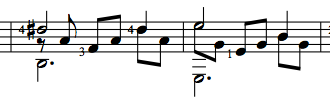In the key of B minor we suddenly get a B7 chord, which doesn’t seem to make sense. However, it resolves to an E minor chord in measure 26. E minor is a temporary “tonic.” So have a fifth above our temporary tonic, E minor, which is B. On that B we have a dominant seventh chord. Because E minor is iv of the key of B minor we would analyze the passage like this.

| V7/iv | iv |

Sometimes secondary dominants are written without the slashes, instead using arrows: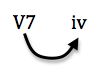Posted on in Music Theory

•